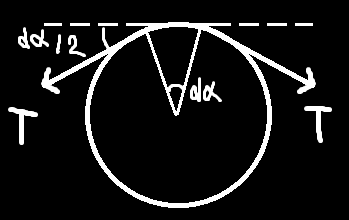# Is my solution correct?

Please tell whether my method is correct or not.
Problem statement: Two blocks are connected over pulley with light thread. The pulley is rough and does’t rotate.
I have taken the angular acceleration of pulley as 0.

you forgot about the friction in the pulley

1 симпатия

how will it effect? could you provide your solution?

there is no rotation so there shouldn’t be any change in tension forces… i think??

There’s a well known formula that when the rope is wrapped at angle \alpha, then

T_2 = T_1 e^{\displaystyle\pm\mu\alpha}

plus-minus depends on the direction of motion

1 симпатия

but how can we derive it here? could you explain please?

The derivation is very similar to the derivation of Laplace pressure formula. We take a very small part of the rope which makes a small angle d\alpha.The normal reaction force is N = 2\cdot T\cdot d\alpha/2, and dT = \mu N = \mu Td\alpha. Then you simply integrate it

2 симпатии

Thanks!

I have got the answer as this :

If we put in values, m1 = 2/3 kg, m2 = 8 kg, µ = ln(2)/π. I am getting 50/7 m/s^2. is this correct?

yes, it’s right

1 симпатия

Oh thanks!